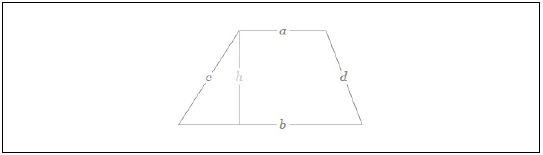# Java Program to Find the Area of a Trapezium

In this article, we will understand how to find the area of a trapezium. Trapezium is a type of quadrilateral that has at least one pair of side parallel to each other. The parallel sides of a trapezium are called bases and the non-parallel sides of a trapezium are called legs. It is also called a trapezoid.

The area of a trapezium is calculated using the formula −

(height/2 * (side_1 + side_2).
i.e.
Area = ½ x (sum of the lengths of the parallel sides) x perpendicular distance between parallel
sides

Below is a demonstration of the same. Area of a trapezium with the length of the parallel sides a and b and the height of the trapezium h is given by −Input

Suppose our input is −

side_1 = 5
side_2 = 6
height = 6

Output

The desired output would be −

Area of trapezium is: 33.0


## Algorithm

Step 1 - START
Step 2 – Declare three integer values namely side_1 , side_2 and height. Declare a float value
namely my_area.
Step 3 - Read the required values from the user/ define the values
Step 4 – Calculate the area of the trapezium using the formula (height/2 * (side_1 + side_2)
and store the result
Step 5- Display the result
Step 6- Stop

## Example 1

Here, the input is being entered by the user based on a prompt. You can try this example live in ourcoding ground tool.

import java.util.Scanner;
public class AreaOfTrapezium {
public static void main(String args[]){
int side_1 , side_2 , height ;
System.out.println("Required packages have been imported");
Scanner my_scanner = new Scanner(System.in);
System.out.println("A reader object has been defined ");
System.out.print("Enter the length of the first parallel side: ");
side_1 = my_scanner.nextInt();
System.out.print("Enter the length of the first parallel side : ");
side_2 = my_scanner.nextInt();
System.out.print("Enter the heigth of the trapezium : ");
height = my_scanner.nextInt();
float my_area = (height/2 * (side_1 + side_2));
System.out.println("The area of trapezium is: " + my_area);
}
}

## Output

Required packages have been imported
A reader object has been defined
Enter the length of the first parallel side: 5
Enter the length of the first parallel side : 6
Enter the heigth of the trapezium : 6
The area of trapezium is: 33.0

## Example 2

Here, the integer has been previously defined, and its value is accessed and displayed on the console.

public class AreaOfTrapezium {
public static void main(String args[]){
int side_1 = 5, side_2 = 6, height = 6;
System.out.println("The sides and height of the trapezium is defined as " +side_1 + ", " + side_2 + " and " + height);
float my_area = (height/2 * (side_1 + side_2));
System.out.println("The area of Trapezium is: " + my_area);
}
}

## Output

The sides and height of the trapezium is defined as 5, 6 and 6
The area of Trapezium is: 33.0